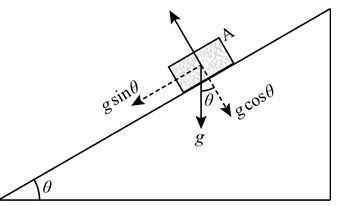×#### Thank you for registering.

One of our academic counsellors will contact you within 1 working day.

Click to Chat

1800-1023-196

+91-120-4616500

CART 0

• 0

MY CART (5)

Use Coupon: CART20 and get 20% off on all online Study Material

ITEM
DETAILS
MRP
DISCOUNT
FINAL PRICE
Total Price: Rs.

There are no items in this cart.
Continue Shopping
```
Two blocks are at the top of an inclined ramp. Block A slides down the ramp without friction; block B falls vertically without friction at the same instant. (a) Which block reaches the bottom first? (A) Block A (B) Block B (C) They arrive at the same time. (D) They is not enough information to answer the question. (b) Which block reaches the bottom with the larger speed? (A) Block A (B) Block B (C) They arrive with the same speed. (D) There is not enough information of answer the question. (e) Which block experiences the larger acceleration? (A) Block A (B) Block B (C) They experience the same acceleration. (D) There is not enough information to answer the question.

```
5 years ago

```							(a) The correct option is (B) Block B.Below figure shows the block A slides down the ramp without the friction. The acceleration of A block is vertical component g (g cosθ) which is always less than the value of g.Since the block B falls vertically without friction at the same instant, so the acceleration of the block B will be equal to the acceleration due to gravity g.From the above observation, acceleration of the B block is greater than the acceleration of A block. Therefore block B reaches the bottom first. Thus option (B) is correct.(b) The correct option is (C) they arrive with the same speed.From equation of motion we know that,v2 = u2+2gh …… (1)Where u is the initial speed of the object, v is the final speed of the object and g is the acceleration due to gravity.Initial speed of both the object is zero (u=0).Substitute initial speed of both the object (u=0) in the equation (1), then equation (1) reduces to,v2 = u2+2gh = 0+2ghSo, v = √2gh …… (2)Since for both the object the value of acceleration due to gravity g and height h from where they fall are equal, therefore from equation (2) we observed that the speed at which both the block reaches at the bottom will be same.Thus option (C) is correct.(c) The correct option is (B) block B.Below figure shows the block A slides down the ramp without the friction. The acceleration of A block is vertical component g (g cosθ) which is always less than the value of g.Since the block B falls vertically without friction at the same instant, so the acceleration of the block B will be equal to the acceleration due to gravity g.From the above observation, acceleration of the B block is greater than the acceleration of A block. Thus option (B) is correct.
```
5 years ago
Think You Can Provide A Better Answer ?

## Other Related Questions on Mechanics

View all Questions »### Course Features

• 101 Video Lectures
• Revision Notes
• Previous Year Papers
• Mind Map
• Study Planner
• NCERT Solutions
• Discussion Forum
• Test paper with Video Solution### Course Features

• 110 Video Lectures
• Revision Notes
• Test paper with Video Solution
• Mind Map
• Study Planner
• NCERT Solutions
• Discussion Forum
• Previous Year Exam Questions